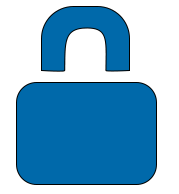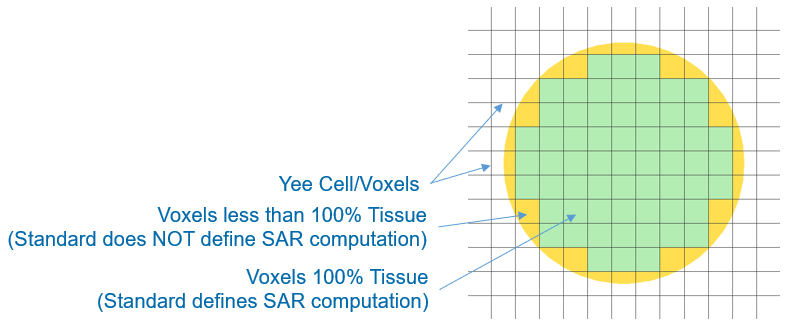# Accurate & Consistent SAR Results | XFdtdRecommendations for grid alignment.

XFdtd's algorithm for computing specific absorption rate (SAR) is derived from and verified against the international IEC/IEEE 62704-1 standard , which requires that dielectric volume averaging be enabled on the tissue material part and SAR be defined only on voxels consisting entirely of tissue. These requirements exclude some cases, which are addressed by additional guidelines for applying the standard using XF.

XF project setup recommendations help users generate a computational grid in cases involving partially filled voxels in order to obtain consistent, accurate SAR values.

Two corrective actions may help resolve project issues:

• Enabling dielectric volume averaging.
• Aligning the grid line with the tissue material's surface.

## Specific Absorption Rate

Raw SAR is defined at a point as

\begin{equation} SAR = \frac{\sigma |E|^2}{2\rho} \end{equation}

where $\sigma$ is the electrical conductivity, $E$ is the complex electric field, and $\rho$ is the density.

In a discretized computational space, raw SAR is defined at the center of each regular cuboid, or voxel, using the values $\sigma$, $\rho$, and $E$. The raw SAR values and corresponding voxel densities are then post-processed for one-gram and ten-gram averaged SAR values.

In discretized computational space for the finite-difference time-domain (FDTD) method, SAR is based on voxels defined by the Yee cells.

## IEC/IEEE Standard Considerations

The standard sets the following regulations on SAR usage:

• Dielectric volume averaging must be enabled on the tissue material part.
• SAR is computed on voxels.
• SAR is only defined for voxels that have tissue materials on all sides.Enabling dielectric volume averaging on the tissue material part affects how the fields are computed during the simulation, but does not affect the definition, density, or voxel conductivity of the SAR computation.

SAR is only computed on voxels consisting entirely of tissue. When a tissue material is not completely aligned with the Yee cells, such as a cylinder discretized by a uniform grid, the partial tissue voxels are not addressed by the standard and are excluded from XF's SAR computations.

## XF Project Setup

XF's grid defines the FDTD Yee cells, which in turn define the voxels. XF's computational grid creates the twelve Yee cell (voxel) edges, from which the complex electric field, $E$, is derived. This value is used to locate the raw SAR value at the middle of the voxel. Consistent, accurate SAR values are therefore dependent upon a grid that accurately represents the geometry, particularly at the surface and near-surface regions where SAR is the highest.